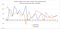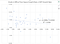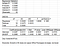# The Requirement of Stationarity in Time Series Regressions

Multiple regression is very commonly used not only in academic research, but also in governments’ policy studies. For example, in one of the consultancy reports for the HK2030+ consultation (about land use planning of Hong Kong), ICF (2017) conducted a review of land requirement for Grade A Offices, Business and Industrial Uses by means of an Econometric Model based on the estimation results of a Time Series Regression.

The report reports that the econometric estimation of Grade A office demand is based on the GDP growth rates of Hong Kong and the difference of GDP growth rates of Guangdong, Mathematically

However, the report directly applies the econometric model for predicting the future demand of Grade A office space, without mentioning the time series regression estimation of the coefficients by means of the past data.

Furthermore, since the econometric model is based on a time series regression, a stationarity test is necessary before carrying out the regression analysis. A stationarity test of the variables is required because Granger and Newbold (1974) found that regression models for non-stationary variables give spurious results.

For example, Figure 2 shows the total quantity of Grade A Office Floor Space (OFS) and Real GDP of HK (GDP) from 1985 to 2017, both have been increasing due to their cumulative nature. Thus, the positive relationship between the two series estimated by a regression model may be spurious.

Figure 3 shows the simple regression results of OFS and GDP, and the Adjusted R-squared is unreasonably high at almost 93%, and they are found to exhibit a positive and significant coefficient of about 2.94. If the results are taken, then the prediction of future Grade A office demand may be mis-estimated by multiplying the Real GDP of Hong Kong by 2.94.

Since both series are increasing, i.e. non-stationary, they have to be converted into stationary series before carrying out regression analysis. Taking first-difference and second-difference are the most common methods to convert a non-stationary series into a stationary one.

Figure 4 shows the first-difference of the natural logarithm of the two series (to represent the yoy growth rates), but the stationarity of the variables is not guaranteed. Stationarity of time series is commonly tested by Dickey-Fuller of Augmented Dickey-Fuller Unit Root Test.Figure 4 First-difference of the natural logarithm of OFS and GDP (yoy growth rates), 1986–2017

For illustration sake, the data series of the dependent variable: dln(OFS) and one of the independent variables: dln(GDP) are tested and the results are shown as follows: both series are found to be non-stationary even after taking the first-difference at the 5% level. (Figures 5 and 6) Thus, any regression estimations between dln(OFS) and dln(GDP) may be spurious and should not be used.

Furthermore, a regression analysis of dln(OFS) and dln(GDP) found a NEGATIVE relationship between the two, probably due to the time lagging effects of GDP on OFS. Yet, the consultancy report does not address about the time lags in the econometric model, but assuming a positive coefficient. The negative relationship may produce an implausible result that a higher GDP growth rate would cause a lower OFS growth rate. (Figures 7 and 8)Figure 7 The Scatter Plot shows a Negative Relationship between dln(OFS) and dln(GDP)Figure 8 Regression results of dln(OFS) and dln(GDP) shows a negative relationship

Thus, there are two potential flaws in the consultancy report on the review of land requirements for Grade A office demand. First, the series are not stationary. Second, the relationship between the growth rate of Grade A office space and the growth rate of GDP is empirically found to be negative in Hong Kong, probably due to the missing time lagging effect. Assuming a positive coefficient is however not supported by empirical evidence.

References

Granger, C.W.J. and Newbold, P. (1974) Spurious Regressions in Econometrics, Journal of Econometrics 2, 111–120. https://wolfweb.unr.edu/homepage/zal/STAT758/Granger_Newbold_1974.pdf

ICF (2017) Final Consultancy Report for HK2030+, Review of land requirement for Grade A Offices, Business and Industrial Uses, January, Job #: J60300046, ICF Consulting Services Hong Kong Limited. https://www.hk2030plus.hk/document/Review%20of%20Land%20Requirement%20for%20Grade%20A%20Offices_Business%20and%20Industrial%20Uses(Eng)_FCR.pdf

Written by